October 31, 2006

Logarithms Homework

(1) Solve for x:
(a) log8x = 2
(b) log4x = -(3/2)
(c) logx27 = (3/2)
(d) logx5 = -(1/2)

(2) Expand:
(a) log2M5N4
(b) log2(M4/N3)

(3) Given that log109 = 0.95 and log102 = 0.30, without using a calculator evaluate:
(a) log1081
(b) log103
(c) log10(9/2)
(d) log10(1/2000)

scroll way down for the answers ....

don't look until you're tried all the questions ....

HEY .... no peeking!!!!!! ;-)

Wonder what this picture has to do with logarithms ....... something ... can you figure out what? ;-)

(1) (a) 64 (b) 1/8 (c) 9 (d) 1/25
(2) (a) 5log2M + 4log2N (b) you figure it out. ;-)
(3) (a) 1.9 (b) 0.475 (c) 0.65 (d) -3.30

Exponentials & Logarithms --a trick for students; a treat for teachersAt the beggining of class we were given 9 questions to solve they were seen on the whiteboard like this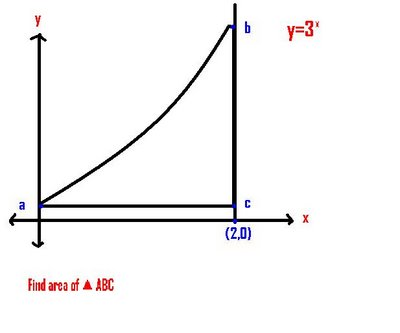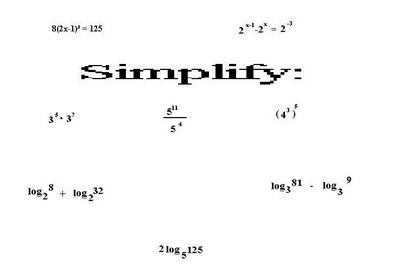We were given time to solve this equations, after the time was done we explained how we got our answers.

Question #1

To find the area of triangle ABC we had to find the points of A, B ,C is this is the step by step solution.

Point A is found by plugging our x value into the equation y=3x, our given value for x was zero(0), and any power to the exponent of 0 will always equal to 1, which gives us the coordinates (0,1) and that is the coordinate for point A

Point B is found by plugging in our x value also into the equation, we got the value of x by the ordered pair below point B which was (2,0), when we plug in the value of x into the equation y=9, so the coordinates of point B would be (2,9)

Point C is found because we are given the x value which is also seen by above in Point B, and the point Below it, because there on the same x distance, but just different y distance,so the x value is 2, and the y distance is going to be the same as point A because it lies on the same height as point A, so the y value is 1, so the coordinates of Point C is (2,1)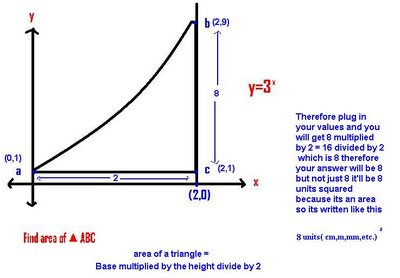So the solution for question #1 is 8 units squared

Question #2

the solution to question # 2 is found by finding the value of base of x, we simplified it into becoming 2³(2x-1)³=5³, it had the same exponents so we dropped them and simplified it to [2 (2x-1)] ³ = 5³, then we simplified it by it equaling 4x-2 =5, we then moved the -2 onto the other side od the equal sign and we get 4x=7, we then isolate the x and get x= 7/4

So the solution to question # 2 is x=7/4

Question #3

It was a homework question for two classes ago. First off we went by re-writing the question into making it look like this 2^x/ 2 - 2^ x= 2^-3, to make the fraction disappear in the equation we multiply by 2 on both sides of the equal sign, therefore the equation will now look liek this 2^x - (2)2^x=(2)2^-3, at this point dont add because you'll end up getting the original equation, so what you do now is solve by factoring. You will isolate the varaible 2^x. So it will be written like this 2^x(1-2)=2^-2, it will then end up like -2^x = 2^-2 , after simplifying. Therefore we concluded there is no solution because even if you were to multiply everything by 1 youll still have a postive power one side, and a negative power on the other, therefore staing its not true.

So the solution for question # 3 is NO SOLUTION

Question #4

When we were asked to simplify (3^ 5)(3^7), we can say that when we are multiplying powers with the same base we add the exponents, therefore it can be simplified by writing it like this 3^(5 +7), in other words 3^12

So the solution for Question # 4 is 3^12.

Question # 5

when we are asked to simplify 5^11/5^4 we can say that when dividing powers with the same base we subtract exponents. If you apply this concept you will get an outcome like this 5^(11-4) or in other words 5^ 7.

So the solution for question #5 is 5^7

Question # 6

When we are asked to simplify (4^3)^5, we can say that when a power is raised to a power we multiply the exponents, so it would look like this [4^(3)(5)] or in other words 4^ 15

So the solution to Question#6 is 4^15

Question 7-9 remember LOGARITHMS ARE EXPONENTS!! BECAUSE MR.K KNOWS THAT AT LEAST 6 OF US ARE GOING TO FORGET. These questions are also for us to adapt on knowing how to run our tools backwards, and fowards.

Question#7

When asked to simplify log2^8 + log2^32, we look at as its adding expoents, so your multiplying powers that have the same bases, so that question can be simplified as log2^(8)(32) or log2^256.

So the solution for Question #7 is Log 2^256

Question #8

When asked to simplify log3^81 - log3^9, we look at it as we are subtracting exponents meaning we are diving powers. Thefore the equation can be written like log3^(81/9) or in other words log3^9.

So the solution for Question # 8 is log 3^9

Question #9

When asked to simplify 2log5^125, we see here that we are going to multiply powers, which can be also known as to raising a power to a power. So it can be written like log5^125^2,or in other words log 5^15625

So the solution for question #9 is log 5^15625.

log a^(mn) = log a ^m + log a^n

log a ^(m/n)= log a ^m - log a^ n

log a^(m^c) = C loga^m

The above was written on the whiteboard after the qesutions were completed. These are the concepts we applied to our questions from earlier to get our solutions.

We were then given some new questions, and we were asked to write them as a single logarithm

The four questions were

a) 1/2 log a^9 - log a^3

simplify the equation as log a^3 - log a^3 which will give us loga ^ 3/3 by the corresponding concept of subtracting exponents meaning your dividing powers, you will then get to simplify as loga^1 which will give us 0.

The solution to a) is zero(0)

b)log a^6 + log a^4

adding exponents is the same as multiplying powers, so therefore it would simplify as loga^(6)(4), which in other words can be loga^24.

The solution to b) is loga^24

c) 2log a^5 + loga^4

simplified as loga^25 + loga^4, then we apply the adding exponents meaning multiplying powers so we simplify it by writing it as log a^ 100.

The solution to c) is loga^100

d) log a^ 6 + log a^ 8 - 1/2log a^16

therefore we simplify the adding of exponents as multiplying powers, and then simplifying the multiplying of exponents as power raised to a power, so it can be rewritten like,loga^(48)-log a^(16^1/2), which then can be simplified as loga^ (48/4), which can even be more simplified as loga^12.

The solution to d) is log a^12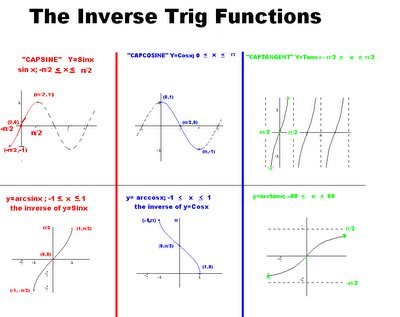NEW PAGE EVERYBODY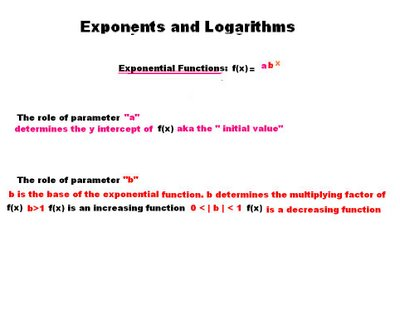Logarithms

Definition:

#1 the logarithm function is the inverse of the exponental function.

#2 the logarithm function is the function that turns powers into exponents.

REMEMBER: A LOGARITHM IS AN EXPONENT!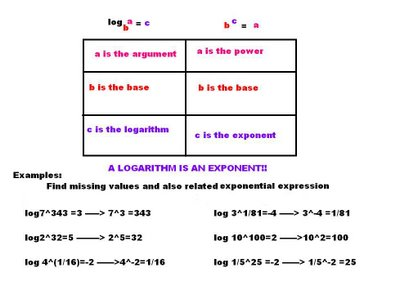And thats all for today folks.....and the next scribe is Richard..he knows already =)

October 30, 2006

Jefferson's scribe post - brief intro to logs

We were given questions as usual:

Sketch:

a) f(x) = sinx on the interval [-pi/2, pi/2]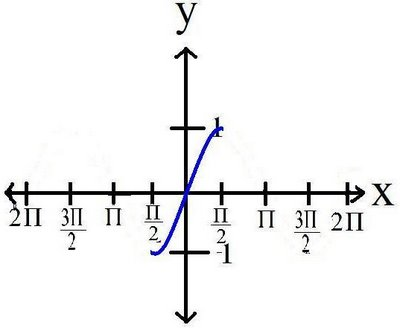*sketch a normal sine function to start.
since there was a domain given erase the graph so that the sine function is between -[pi/2 and pi/2]

a) g(x) = cosx on the interval [0, pi]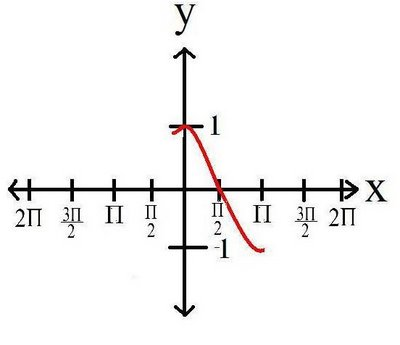*pretty much the same as the top

c)h(x) = tanx on the interval [-pi/2, pi/2]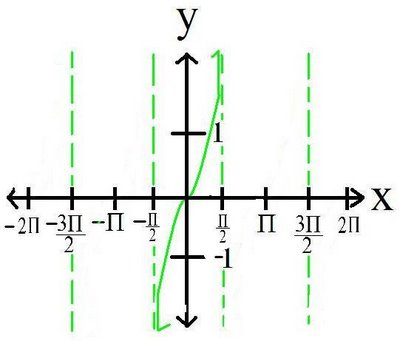*and again the same steps apply here

next we were asked to sketch the inverse of the three graphs

a)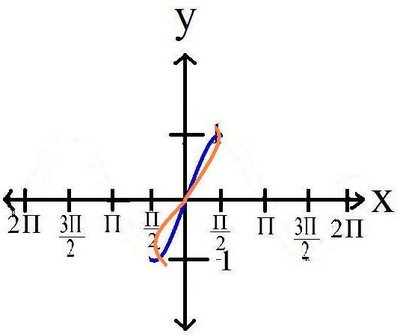*draw the line of reflection y=x.
the coordinates change from (x,y) to (y,x) ei (2,3) to (3,2)
the original graph is just flipped over the line of reflection

b)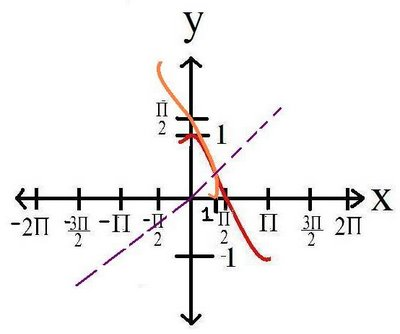*same rules apply

c)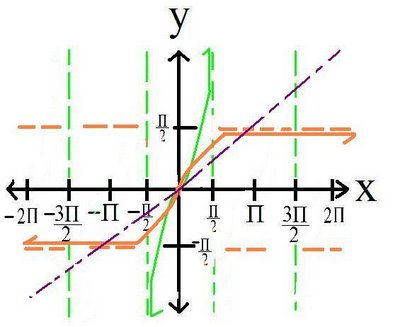*this one may be a little tricky
draw the line of reflection to start out with
since the graph is being reflected, so are the asymptotes
the asymptotes are now at x = 0 (orange)

Next we were asked to sketch:

y = 2^x

*the two most easy points you can get are when you input 0 and 1. If you replace x with 0 as an input you get an output (y coord) as 1. if you replace x with 1 as an input, then you get an output of 2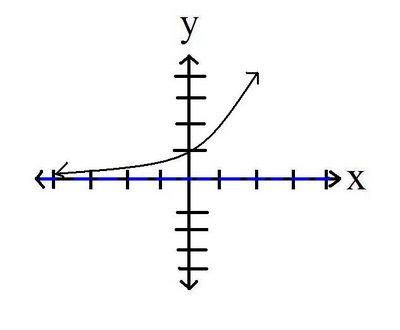y = 3^x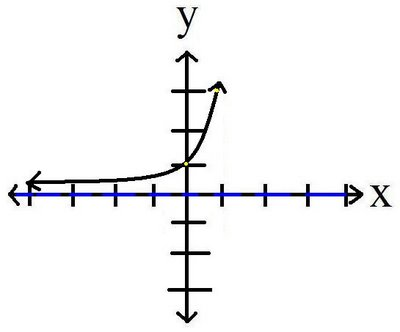next we had to sketch the inverse of the two :
*draw the line of reflection first. since the graph is being flipped over the line y = x, the asymptote(blue) is also being flipped.
*the new asymptote is at y = 0 (seen in red)
*if you forget the order of pairs (x,y) are flipped to (y,x)

y = 1/(2^x)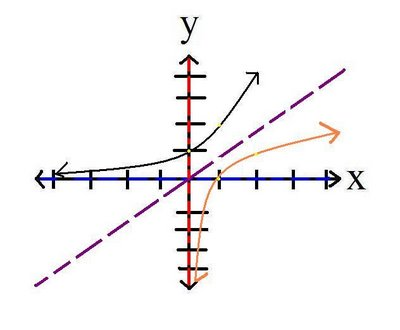y = 1/(3^x)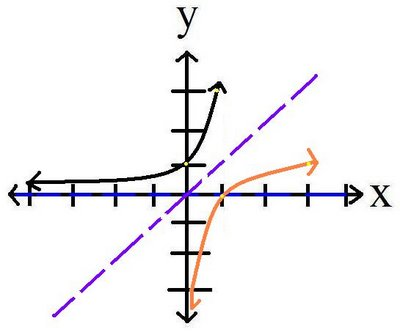when you input a number you nonetheless gt an output of the result. the inputs of these graphs are the exponents(x). And from the input you get an output of a power. IE 2^2(input/exponent) = 4(output/power)

Now when you do the inverse, the input is the power and the output is the exponent. it's basically saying it backwards, that's pretty much what an inverse is...sorta.

NOW it is time for me to bring in the logarithm aka the LOG(no not the tree log) . a log is a number but not any number. its a number that is formed from a result. An example Will come up later on this scribe post.

ba=c

b = base
a = exponent
c = power

this is the basic formula for powers and exponents

a logarithm reverses that equation:

logb(c) = a

this equation says that a logarithm is an EXPONENT!
you WILL i repeat WILL hear Mr k repeat this 100 times! because students will often forget about this

here's an example:

23 = 8
log2(8) = 3

*the 8 and 3 switch because a logarithm is an exponent.

heres another
53 = 125

log5125= 3

the next scribe will be.....jhoann

October 27, 2006

Scribe Post

The morning was nothing new since we had a test the entire period, so there was one or two new bits of information during the afternoon class.

When we first came in the usual questions were on the board.

Solve for x:
8^x=32
2^3x=2^5
3x=5
x=5/3
3-3^x-1=1/27
3^1+(x-1)=3^-3
1+x-1=-3
x=-3
5x^4=80
x^4=16
(x^4)^1/4=(16)^1/4
x=2
1/4^x-2=64
4^-x+2=4^3
-x+2=3
-x=1
x=-1
2(5^2x-9)=250
5^2x-9=125
5^2x-9=5^3
2x-9=3
2x=12
x=6

There was one last question which gave people plenty of problems since almost everyone was apparently making the same mistake in the solving.

2^2x=3(2^x)-2
(2^x)^2-3(2^x)+2=0
LET a = 2x
a^2-3a+2=0
(a-1)(a-2)=0
a=1 or a=2
~~~~~~~~~~~~~~~~~~~
2^x=1
2^2=2^0
x=0
~~~~~~~~~~~~~~~~~~~
2^x=2
x=1

The common mistake that people were making was doing something along these lines:

2^2x=3(2^x)-2

For the next step they would divide both sides by 2^x but did not cancel the -2 at the end of the equation. This cannot work and was explained to us why in this example.

3+7
----- = 7
3

If we were to follow the idea above then only the 3 would cancel and the answer would be 7 instead of the proper answer being 10/3

We also learned about some other minor bits of information but it's not really important as it was more about some of the graphing calculator functions.

The next scribe on Monday will be Jefferson.

Exponential Equations Homework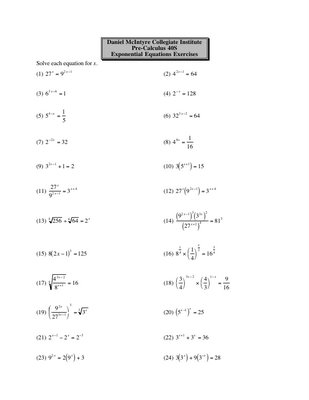October 26, 2006

Scribe Post:Exponents and finding other ways to write a number using exponents

Hi it's Ashley and I'm the scribe for today!
In class Mr.K wrote down 5 numbers on the board and told us to come up with 4 different ways to write down the number using exponents. The numbers were 2, 4, 3, 1/2 and 4/9. Seems hard huh? But don't worry once you see the pattern it gets easier.
By the way for those that don't know, this symbol ^ means to the power of. So for example 2^4 means 2 to the power of 4 which equals 16.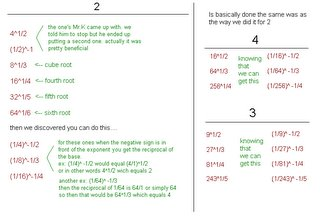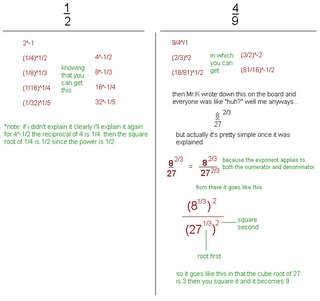*Remember that there infinite ways to find another way to write a number.*
Then Mr.K said that knowing how to do that you can do this. I'm pretty sure that's what he said.
2^x=32 (well remember 32 can be written as 2^5)
2^x= 2^5
x=5
3^x-1 = 27 (find a way using the number to find the same base, in the case 27 can be written as 3^3)
x-1 = 3
x=4

In these questions like the questions above you are asked to prove and find the value of x. The key to these questions is that the idea is to find another way to write the number using a useful base. What you want is to look for a common base for both expressions. The reason why you look for a common base is because if the bases are the same then the exponents must equal the same. Then you just solve for x. It will be further understood when you see the questions below since I'm poor at explaining -_- Oh but the questions might look a little weird since it's hard to write exponents on the computer so here's an example of how it looks like on paper: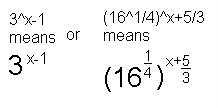1) 2^3-2x = 1/4^x-3/2
the next step is to find another way to write the number using a useful base. in this case we want the base 1/4 to be 2.
2^3-2x = (2^-1/2)^x-3/2 (1/4 equals 2^-1/2)
2^3-2x = 2^(-x/2+3/4) (what you do here is multiply -1/2 to x-3/2 which gives you -x/2+3/4)
now that you have the same base for both expressions you can now solve for x.
3-2x = -x/2+3/4
4(3-2x) = (-x/2+3/4)4 (you want to get rid of the denominator which is 4 so you multipy both sides by 4)
12-8x = -2x+3
12-3 = -2+8
9 = 6x
3/2 = x
2) 3^4x-1 = 27^2x
Like in the previous question you want to find another way to write the number so it can have a common base
3^4x-1 = (3^3)^2x (27 equals 3^3)
3^4x-1 = 3^6x
now sove for x
4x-1 = 6x
-1 = 2x
-1/2 = x

The question below is a little bit trickier if you don't know the laws of exponents. So remember that if you multiply powers with the same base then you add. If you divide powers with the same base then you subtract.
3) (3^x)(27) = 81^2x+1
now look for another way to write the numbers using a useful base. for this question a useful base would be 3 since both 27 and 81 are multiples of 3.
(3^x)(3^3) = (3^4)^2x+1 (27 equals 3^3 and 81 equals 3^4)
*now remember when you multiply powers with the same base you add
3^x+3 = 3^8x+4 (3^x*3^3 the power x and 3 are added together so they become x+3)
solve for x:
x+3 = 8x+4
3-4 = 8x-x
-1 = 7x
-1/7 = x

And just when I thought class was over Mr.K had to put up two more questions on the board. Gheez....
4^2x-4 = 1
like in previous questions rewrite the base so that both numbers have the same base. at first some would think of changing the base 4 into base 1 by having the power be 0 but then the 0 would cancel the whole exponent. so instead you should do this:
4^2x-4 = 4^0 (the 4^0 would equal 1 since any number to the power of 0 expect 0 itself equals 1)
then you go on from there solving x
2x-4 = 0
2x = 4
x = 4/2
x = 2

For this question at first it looks hard but actually what you do is balance the equation first so you can get the power by itself like this:
5^(2x-1)+2 = 3
5^(2x-1) = 3-2
5^(2x-1) = 1

next step is similar to the previous question in that you re-write the 1 to be the same base as 5 by having the power be 0.
5^(2x-1) = 5^0
2x-1 = 0
2x = 1
x = 1/2

So this is the end of my post and if there are any mistakes just comment. Also if it's hard to read sorry about that but for some reason my spacings didn't turn out right. I wonder why it does that? By the way the homework is Ex.20. Apparently if you understand what we did in class the homework will be super easy but remember we still have the test on Trigonometric Identities tomorrow guys so study hard!! Mr. K said the last page of the test will have the identities on it so that's a relief. Good luck everybody!
the next scribe will be Jason!

Oliver's Blogging on Blogging

Okay dokes, this is my blogging on blogging, and I would like to say the trigonometric identities unit was great, I thought it was a lot of fun. I wasn't the best at it but I mean, I just like those kinds of things. I got it at times, and I had some questions at times, but I believe I will do okay tomorrow for the test, and I hope EVERYONE DOES GOOD TOMORROW ! don't forget to study guys ! that is all, bye bye.

JessicaJill's Blogging on Blogging

Hopefully I'm not too late to put in my BOB. I have to admit, trig identities is a tough unit. In the terms of Mr. K, I think I would be better prepared if I had just taken the chance and fall off my bike a couple more times. It's not like I've given up on it, I know I could've studied more to have a little more knowledge to take along with me to test day. Looks like I'm hitting the books. Good luck to everyone.

ruschev's Blogging on Blogging

this unit about trigonometric identities was, at first, very confusing but it all turned out OK! this unit was OK.....good luck on the test tomorrow

Jho-Ann's BOB =)

Trig identities was an OK topic for me for me. I especially like the double angle identities and i don't know why. Fellow classmates think I'm weird cause they say its harder. Though how Mr. K got the sin and cosine stuck in our heads by the weird dance, i think it was pretty clever, because i have it stuck in my head. Ha ha and to tell you did we ever LOOK WEIRD!! I probably like this unit better than the rest. I guess because i was exposed to it more than the other units. The test is tomorrow and i hope i do better than average. Like the bicycle speech we had, i wanna RULE. Not only in this unit but in every other unit as well. I hope everyone does well for tomorrows unit test.

Tennyson's BOB # 3

The subject for this topic was pretty OK , i like hate it when you have to prove identities , they are not easy. Tell you the true i really don't like this unit. But i still trying. Just need more practices. How you explain it was very good , i understand it more this year than last year.Well i hope i do good on the test ,but i have a feeling that I'm not going to get a high mark. I guess the thing about identities is that you have lots of ways to do it not just one. So, what you think you got wrong , actually is right.other wise yeah. Bye

Lisa's Blogging on Blogging #3

Oh boy, i hope this isn't too late... i was told today that the test is on friday, which means i still have some time to finish this... i wrote BOB on my hand but didn't see it until now... well this unit seemed pretty easy in the beginning... i found the first equations super simple to solve and follow... but as we got harder and harder my obvious troubles with equations seemed to creep up again... and when i lose faith in my knowledge, i lose all my composure and can't solve a thing... even if i do know my stuff deep down... my math expertise has really fizzled and now i need to pay attention more then ever... i think the hardest part for me is putting it all together... for example i may know my stuff, but when its phrased differently then i'm use to i can't seem to figure it out even if its the exact same question... like the pre-test my brain just said "i don't know what its talking about" and i couldn't figure it out... but when it was explained i was like "oh hey that's just like this!!".....i think i really need to just keep getting up on that bicycle and trying again no matter how many times i fall.... i'm doing my best, but my best isn't good enough so i have to try harder... and i will.... i guess that's all for now i gotta be getting to bed.... Night all!!!

October 25, 2006

Ashley's BOB part3

i think i'm going ok on trigonometric identites although the pre-test was hard. for me anyways because sometimes i don't understand the question and sometimes i don't know how to solve it but i'm understanding now. i think. i just need to practice lots especially on the proving part because i get stuck a lot and i need to start recognizing the identites too but i sort of understand double angles now. so the test is on friday. good luck guys!

BOB

Identites...I'm pretty sure I understand the concept. The only thing that's giving me somewhat a hard time is memorizing the double angle identities. The pre-test for me was pretty straight forward until the last question where we had to prove the identity because I didn't know what could be substituted in for the cos2θ. My pre-test group did an awsome job though...good luck everyone =)

JessiccaI_198's BOB for Identities

I have to say that this unit was confusing at first. When I learned it, it was all right, I understood it. Then there were rules like the sine/cosine dance we did, as well as several double angle identies. There could be so many different ways to solve an equation and sometimes it was hard for me to figure out how to do it. I can memorize the identities, but I have a bit of a hard time applying them to different problems. This is really similar to algebra to me, but with more variables. Until the test, I'm going to try and focus on getting help and doing some of the problems I don't know.

Let's just get on with the scribe....in the morning we had a pre-test on our unit on Identities. The questions will be posted below as a tool to help you study.

1) Simplify: sin(2x + π)

(A) sin2x (B) cos2x (C) -2sinx (D) -cosx

2) The terminal arm of angle θ in standard position passes through point (m,n), where m>0, n>0. Determine the value of sin (π + θ)

(1) (c)

(2) (a)

(3) 1

(4) π

(5) should end up with tanx=tanx

In the afternoon class we started our new unit.

Complete the table of values:
Then we completed this question:
-2^x
(-2)^x The graph of this function has no y-value for all the odd numbers because you can't take the square root of a negative number.
These two are not the same things.
HOMEWORK: ex. 19
next scribe is Ashley =)

October 24, 2006

James' Blogging on Blogging - Part 3

Pre-test tomorrow... I don't know if I am ready or not, I know how to apply everything, it's just i can't identify it right. I know this unit gave me a hard time last year also. Looking forward to the next unit :P I'll finish up my little BoB here.

Good luck tomorrow everyone!

- James

Jess' Blogging on Blogging # 3

So at first, i understand this unit very well, and i think it was pretty much easier than the other units, but lately, i haven't been to class so i missed out some lessons and i feel like i get really behind, but hopefully, in two days before the test, ill understand and know what to do, especially with those tricky questions!
Anyways, wish all of you guys will ace the test :)

Richard's Blogging on Blogging 3

TRIGONOMETRIC IDENTITIES

I guess this is the easiest unit so far. Maybe because I like Algebra and it's fun to untangle things out. It's just like a mystery that you need to solve using a lot of proven data. Aside from that, there is a lot of possible ways to use in order to get the answer. You just have to think wide. Think of the other possibilities.

I don't have any difficulties regarding this unit. It is just some of the questions are tricky. You can get it simply in a short way. But if you miss some ideas, it may lead you to a long and tangled equation.

I hope we all do good on our test. Do your best! Don't ever, ever give up!

Blogging on Blogging Part 3

identities, identities, identit..... well it's a good topic to do but i still have some problem on solving them if i start the question out right, later i will get confuse on what do next or which steps would get me there to get the answer. only if had enough time to solve it i may get the answer but practice, practice make some people better at these things ummm..... well goodluck to all and let the good come to you wuhahaha!!!!

Scribe Post !

Trigonometric Identities
Okay everybody, Good evening ! How are you guys? Well, I hope you all are doing fine, this is my second scribe post, and a brief summary of how our math class went was, we had a quiz first thing, corrected it, showed us different ways on how to answer the questions, and also note that, ( those questions in the small quiz were in the old exams, which may come up in our final exams ). After doing our corrections on the quiz, then corrected our math homework, which was done the last night. Mr. K then corrected it, and he spoke fairly fast, it was probably because we were running out of time, because of the corrections made on the quiz.
Well, on the quiz, I felt I knew what I was doing, and when we corrected it, I, for one, had some minor mistakes, and errors, which caused me dearly, because I could of had a higher mark, but yes, I will learn from this mistake which is really the BEST part of learning. One of the questions that seemed tricky to me was number one, it was only tricky because I had not thought about the identities shown to us on the dictionary. The question on the quiz was like this:
Simplify: sin2θ/sinθ, now, by looking at that, do you think you know how to answer it? I bet you do, well here's the answer :

Simplify: sin2θ/sinθ, now from here, automatically you can go straight to 2cosθ, which is the answer. But just to show you how I got it, then here it is, because math, is, FUN!
= sinθcosθ+cosθsinθ/sinθ
= sinθcosθ/sinθ + cosθsinθ/sinθ
The sinθ's reduce, and you are left with 2cosθ, which was told earlier, is the answer.
Now, After we corrected those questions with Mr. K , we then went to our homework from last night, and corrected it with Mr. K. Here are some of the questions and answers from the hoemwork:
2. Simplify: tanθ/cscθ
3. Write an equivalent experession: cos(3x)(2x)+sin(3x)sin(2x)
5. Prove: 1/1-sinθ + 1/ 1+sinθ = 2tan^2θ+2
Question number 2 looks like this:
tanθ/cscθ
=(sinθ/cosθ )/(1/sinθ)
=(sinθ/cosθ)(sinθ/1)
=sin^2θ/cosθ
( now from here, this is the answer, but to make things more interesting it can turn out to this !
=sin^2θ/cosθ
=(sinθ/cosθ)(sinθ)
=tanθsinθ

For question number 3, this was how it was solve:

cos(3x)cos(2x)+sin(3x)sin(2x)
= cosx
(it's as easy as that !)
Finally but not least, the last question:
1/1-sinθ + 1/1+sinθ = 2tan^2+2
=1+sinθ+1-sinθ/ 1-sin^2θ
=2/cos^2θIV>
=2sec^2θ
Now, looking at that, you wonder, how did you get the " +2 ", well have no fear, the answer is here. The answer is, if you can't solve it like that, then go to the other side and try to simplify that and see what you get. This is what we got:
1/1-sinθ + 1/1+sinθ = 2tan^2+2
= 2(tan^2θ+1)
= 2sec^2θ
It's just like that, no hassles or nothing, just a small tad bit of thinking, well actually a lot of thinking, if you haven't seen these kinds of problems yet. Also note that, in those kinds of proving questions do not forget to (*NOT PASS THE WALL OF CHINA) , which is the big long line that seperates the two terms telling you that you cannot move anything from either side.
Now this is oliver_796 ABOUT to sign off, but wait, what's that? I here a .. I hear a .. I hear a .. MESSAGE. *DING-DONG. YES? The message is: PLEASE CONTINUE TO ASK QUESTIONS IN CLASS, BECAUSE IT'S A SURE GOOD WAY TO SHOW THE TEACHER THAT YOU WANT, AND ARE EAGER TO LEARN. BY SHOWING THIS, THE TEACHER WILL THERFORE MAKE YOU LEARN, WHICH IS VERY GREAT. =) , okay dokes? Continue to study with your study groups! Continue to perform random acts of kindness ( quote by Mr. K ) and go out there and learn ! Until next time, Take care everybody.
THE NEXT SCRIBE IS ANH! HAVE FUN!

daphne's BOB part 3

The lesson about identities was easier for me than the past lesson. I found it very interesting because when we first took our exercise, I answered it correctly. It helped me to understand it better when I used to answer the questions in the assignments and it amazed me because most of the questions given in the class are the ones given in the assignment. However, there are times that even though I did the assignment I encountered some difficulties in answering some seatworks but then I asked some of my classmates to help me solved the problem and I thank them for doing such a wonderful thing. On the other hand, Mr. K did explained to us everything in a fully detailed manner, so it also gave the whole class better points to remember in solving identities.
In general, the lesson about identities was a challenge for me because it summarizes everything we took starting from lesson one up to the present lesson. Therefore, there is a need for me and for the others to study more because this can tell whether or not we understood the whole lesson.

October 23, 2006

Blogging on Blogging

New unit and at the very least I'm having an easier time with the work then previous questions, though not by much. During homework, or the quiz today for example I end up making all sorts of tiny mistakes that end up costing me the question. If anything I am going to need practice and plenty of it if I even hope to get good marks for this semester. Overall I'd probably just need some basic explanations and practice.

jessica.'s blogging on blogging; identities.

third bob, wow. hm, the topic identities. so far, i think it's okay. like i know what to do, yet i get stuck because i can't find a way to solve it. but mr. k said that we had to find another point of view to find the answer and there are more than one way of solving the identity. and that's pretty much all i need to focus on, but everything so far - i'm alright with (: goodluck guys.

Blogging on Blogging

Well well well.... Another BOB for me... actually, I enjoy our topic. Sometimes I find the problems easy to answer, sometimes I get stuck... but as Mr. K said... practice is the key of mastering this topic so I definitely need to do lots of practice!

Blogging on Blogging

Well well well.... Another BOB for me... actually, I enjoy our topic. Sometimes I find the problems easy to answer, sometimes I get stuck... but as Mr. K said... practice is the key of mastering this topic so I definitely need to do lots of practice!

Jefferson's blogging on blogging- Identities

Well so far so good for me. Proving identities aren't that difficult to do. However doing questions similar from the practise quiz that we had today was a little hard. I really had to think. I'm ok with solving difference identities. We didn't cover the tangent differences identities yet though. but I'm sure I'll do fine with them if we go over that tomorrow. Overall I think I'll do fine on the test. jut some more practise and review and I'll do well =)

Scribe Post, Trig Identities

Hey you guys, it's JessicaJill, and I was your scribe for today's double class. In the morning we had a five question quiz to do in 5 minutes. Here it is.

1. secΘ - tanΘ is identical to:

a) cosΘ/1 + sinΘ
b) sinΘ/1 + cosΘ
c) secΘtanΘ
d) cotΘcscΘ
e) None of these

SOLUTION: The answer is a. Here's why:

2. 1 - cos2Θ/1 + sinΘ is identical to:

a) cosΘ
b) tanΘ
c) sinΘ
d) cosΘ/1 + sinΘ
e) sinΘ/1 - cosΘ

3. 1- sec2Θ is identical to:

a)-tan2Θ
b)tan2Θ
c)cot2Θ
d)-csc2Θ
e)cos2Θ
We know that tan²θ + 1 = sec²θ, given by the pythagorean identity. By switching things around we can get that tan²θ = sec²θ - 1, and that -tan²θ = -sec²θ + 1, which can also be read as -tan²θ = 1 - sec²θ.

4. Given than cosΘ = -1/3 and sinΘ is greater than 0, then sinΘ equals

a) -(2√2)/3

b) (2√2)/3
c) -√10/3
d) √10/3
e) 1/3

5. Given that cscΘ = -4, Θ is greater than π and less than 3π/2, then tanΘ equals

a) √3/4
b) -√15/15
c) -4
d) 4
e) √15/15

Because a majority of the class had a little difficulty with these questions, it wasn't worth marks.

Then we were given three questions on the board to solve: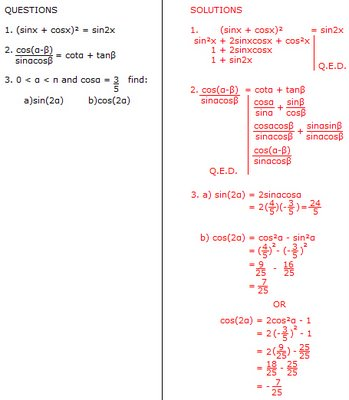After that, he asked us what quadrant cos2α was in from question 3, and we all had different answers. A lot of us were a little afraid of being wrong, so he put us in groups to think about what quadrant we thought it was in. With several different answers given, we came to the conclusion that it was in quadrant III. If you think about what our answer was, where's it's negative and where the related angle is, the answer is clear.

In our afternoon class we got some notes to put into our math dictionaries.

We then were given 5 questions on the board, all that were on (a) previous exam(s), but didn't have enough time to go through the answers in class, so we were told to do it for homework.

QUESTIONS

1. Solve for θER in radians to 3 decimal places [calculator allowed]:

4cos(2θ) + 3 = 0

2. Simplify tanθ/cscθ

3. α and β are quadrant II angles, sinα = 1/3 and sinβ = 2/3. Find the exact value of cos(α + β).

4.Write and equivalent expression: cos(3x)cos(2x) + sin(3x)sin(2x)

5. Prove: (1/1 - sinθ) + (1/1 + sinθ) = 2tan²θ + 2

Well, that's it. Sorry it took me so long to get it up. I had a lot of work in the last week and I wanted everything to be perfect. This post is still a little incomplete, but I wanted it to be published already.

October 20, 2006

Scribe Post for Identities

Hey people! This is Jessicca_198. Today, all we really did was Exercise 18, so there isn't really much for me to inform everyone on. I'm not exactly sure this counts as a scribe post, but the next person to be scribe after me is JessicaJill. Sorry I couln't have anything more for you guys. See you all on Monday!

October 19, 2006

Scribe Post

Hello! This is Jaifel and it's my second scribe post. When we entered the class, Mr. K asked us to find the exact value of :

a. sin 100° cos 50°+ cos 100° sin 50°
we know that this is the sine law

sin (100° + 50°)
sin (150°)
1/2

b. cos π/3 cos π/12 + sin π/3 sin π/12

from our "dance" we know that this is the cosine law

cos (π/3- cos π/12)
and we also know that the sign changes

we then used a common denominator
cos (4π/12- π/12)

and we get (3π/12) we reduce it as cos (π/4)
which has a value of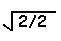He also asked us to prove that ...................

d. cos(π+x)= cosx
cosπ cosx+ sinπsinx
-1 cosx -(0) sinx
-cosx

PROOFS OF THE SUM AND DIFFERENCE IDENTITIES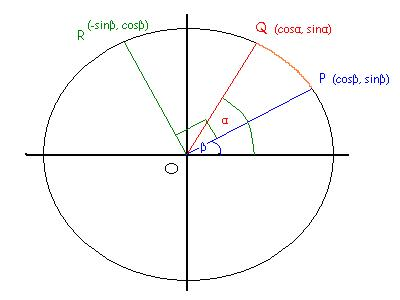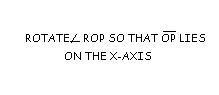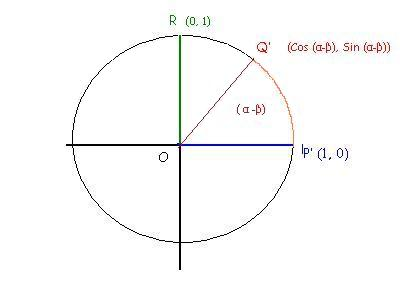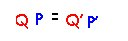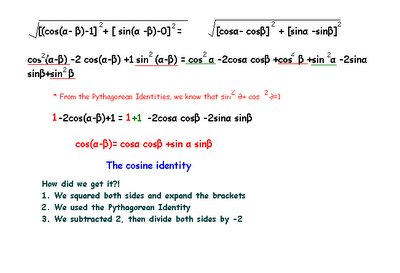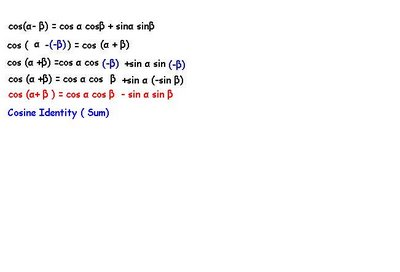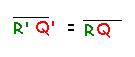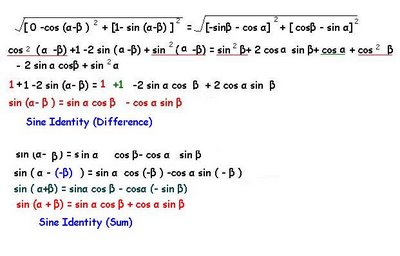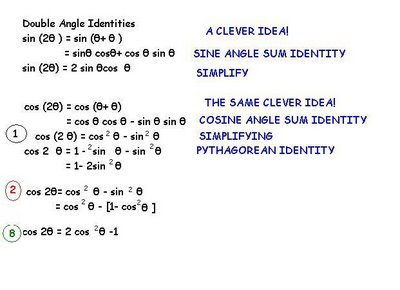Scribe Post

Hi there! This is jaifel and I'm your scribe for this day. It's getting late and I haven't finished my post yet. Anyways tomorrow's scirbe is jessica_198. I'll try to finish my post tonight! owkei dowks! See you guyz at school!

October 18, 2006

Hello everyone. It's me Gerald again and this is my second scribe post. When we entered class, Mr. K put questions on the board for us to do.

QUESTION #1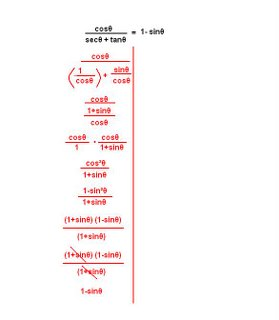SOLUTION IN RED

QUESTION #2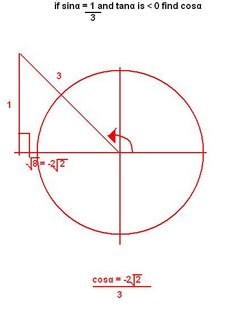SOLUTION IN RED

QUESTION #3
SOLUTION IN RED
OTHER SOLUTIONS MR. K ASKED US TO FIND IN BLUE
towards the end of class, Mr. K came up with a dance for the class to do so that we remember the formula. He made us do the dance 6 times because it takes 6 times for you to go from short term memory to long term memory.
the formula's were:
sin(α+β) = sinα cosβ + cosα sinβ

cos (α+β)= cosα cosβ + sinα sinβ

thats all for today. Its my nap time anyway =) next scribe is jaifel. See you next time

October 17, 2006

Scribe Post, IdentitiesGood evening fellow students, names Steve =o. I hope you guys enjoyed the formulas and the 'plug and solve' methods on previous units because it will be rarely used in this unit, Identities. Like Mr.K mentioned, practice is what will be the key to you mastering these problems, so the beginning of the first class of todays two-class day he threw at us these problems:

Examples & Solutions: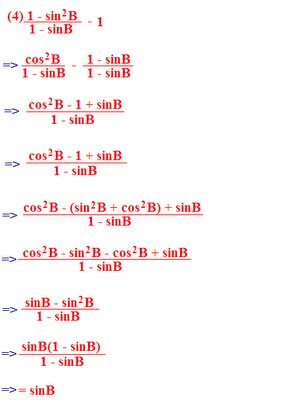Well, goody. Those were the simplification problems, now that they're done with, we have some questions where we have to prove that one operation equals the other. Im kind of running behind schedual, those pictures were hard to put together =P, thats was fun, but lets see if we can deal with simple text typed ones.

Examples: Prove!
1)secx - cosx = sinx+tanx
If starting with the Left side:
=>(1/cosx)-cosx
=>(1/cosx)-(cos2x/cosx)
=>(1-cos2x/cosx)
=>(sin2x/cosx)
=>sinx(sinx/cosx)
=>sinxtanx <,/> <= a check mark =P, squint! You might see it.

If starting with the Right side:
=>sinx(sinx/cosx)
=>(sin2x/cosx)
=>(1-cos2x/cosx)
=>(1/cosx) - (cos2x/cosx)
=>(1/cosx) - cosx
=>secx - cosx <,/>
2)[1/(1-sinx)] - [1/(1+sinx)] = 2tanxsecx
Apparently, we learned an operation would equal another operation if their result is the same, and it DOES NOT have to look like one another, like this example here.
If starting with the Left side:
=>[(1+sinx) - (1-sinx)] / [(1-sinx)(1+sinx)]
=>2sinx/(1-sin2x)
=>2sinx/cos2x <,/>

If starting with the Right side:
=>2(sinx/cosx)(1/cosx)
=>2sinx/cos2x <,/>

Well, that was the end of the first class. It was hard to get used to, so usually alot of explaination was needed to help understand Identities more, which is why we only had 6 questions. Great! you got this far, it's intermission time =P. It was lunch for us, go get a snack or something and come back.Welcome back! Being seated we noticed Mr.K had put up more questions for our... pleasure? Well, lets get to it! Noted, he has raised the bar once more, these were much tougher for us, noticably.

Examples: Prove!
(secx-1)/sin2x = sec2x/(1+secx)
For example: (1/cosx) + 1, representing 1 as (cosx/cosx) is completely legit! This technique can be crucial to figuring out the Identity! As shown in this example.
If starting with the Left side:
=>[(1/cosx)-1]/(1-cos2x)
=>[(1/cosx)-(cosx/cosx)]/(1-cos2x)
=>[(1-cosx)/cosx]/(1-cos2x)
=>[(1-cosx)/cosx] x [1/(1-cos2x)]
=>(1-cosx)/[cosx(1+cosx)(1-cosx)]
=>1/[cosx(1+cosx)] <,/>

If starting with the Right side:
=>(1/cos2x)/[1+(1/cosx)]
=>(1/cos2x)/[(cosx/cosx)+(1/cosx)]
=>(1/cos2x)/[(cosx+1)/cosx)
=>(1/cos2x)/[cosx/(cos2x+1)]

3)[1/(1+sinx)] + [1/(1-sinx)] = 2sec2x
This question is a good example which side is best to pick. Because sometimes a side maybe really hard to figure out, you'll see why in a moment.
If starting with the Left side:
=>[(1-sinx) - (1+sinx)]/[(1+sinx)(1-sinx)]
=>[1-sinx+1+sinx]/(1-sin2x)
=>2(1/cos2x)
=>2sec2x

If starting with the Right side:
=>2(1/cos2x)
=>2/cos2x
Now, this is the part where if people were to pick the right side will be stuck on. Givin the evidence on the left side, 2/cos2x would = [1-sin0+1+sin0]/(1-sin20). Yes that it is true it works out, but would anyone really realize 2/cos2x would = [1-sin0+1+sin0]/(1-sin20) if it havent been for them finding the left hand side first? Meh, *probably* not, so this is a good example why picking the most complicated side first, because you can break it down more easily than building it back up. It makes sense here and in real life too! Is it easier to hop down a cliff (support of course =p) than to climb it?

The last part of the class was inputing stuff into our math Dictionary, enjoy!

Trigonometric Identities
The Fundemental Identities

Tanx = sinx/cosx

Cotx = cosx/sinx

Secx = 1/cosx

Cscx = 1/sinx

The Pythagorean Identities

[sin2x+cos2x=1]]
Corollaries
sin2x=1-cos2x
cos2x=1-sin2x

(sin2x/sin2x) + (cos2x/cos2x) = (1/sin2x)
[1+cot2x = csc2x]
Corollaries
cot2x=csc2x-1
1=csc2x-cot2

(sin2x/cos2x) + (cos2x/cos2x) = (1/cos2x)
[tan2x+1=sec2x]
Corollaries
1=sec2x-tan2x
tan2x=sec2x-1
Even and Odd Identities
sin(-x) = -sinx
cos(-x) = -cosx
tan(-x) = -tanx
Strategies for Solving Trig Identities
(1)Work with the 'more complicated' side first
(2)Rewrite both sides of the equation in terms of sine and cosine
(3)Use Pythagoreal Identities to make appropriate substitution
(4)COmbine Functions
(5)Use Factoring (especially difference of squares)
Well, that's it for my scribe post! Remember, math is the sciences of patterns; And patterns are your key to success in Identities! Your way may be different from your neighbours, but have the same answer. Like Mr.K says:
-Steve, oh right. The next scribe shall be, Gerald. Have fun =]